Next: TclBC Up: User-Defined Forces in NAMD Previous: Introduction

Subsections

TclForces

In this unit, you will learn about NAMD's TclForces functionality. Although many user needs were anticipated in the development of NAMD, to anticipate them all specifically would be impossible. TclForces allows users to easily supplement the built-in constraint and force functionality with script-based forces.

Introduction

The basic idea of tclForces is simple: we provide NAMD with a Tcl script which tells NAMD to apply certain forces to certain atoms. The only special requirement of this script is that it define a command named calcforces. This command is called by NAMD each timestep. Within the script, we can access atomic IDs, positions and masses. In this unit, we will see how to use tclForces through four examples of increasing complexity.

Example 1: Constant Forces

For our first exposure to tclForces, we will do something very simple: apply a constant net force to a system.

1
First, let's look at the NAMD configuration file. Go to the directory forces-tutorial-files/tclForcesFiles/push and open the file push.namd. Everything is standard about it until the end, where we see some unfamiliar lines:

 tclforces on set linaccel "30 0 0" tclforcesscript push.tcl

The first line simply turns tclForces on. The second sets a parameter for the acceleration that we'll use in the script, as we'll soon see. The last line is the name of the script which actually does the work.2
Now let's look at the script. Open the file push.tcl, in the same directory.

3
First, we select the atoms we're interested in:

 set numatoms 1231

 set atoms {} for { set i 1 } { $i <=$numatoms } { incr i } { lappend atoms $i }  foreach atom$atoms { addatom $atom } The structure we're using is ubiquitin, the same protein used in the VMD and NAMD Tutorials. Here, we're using the protein alone, in vacuum, and so the structure contains just 1231 atoms. We first build a list of the atomic indices (NAMD atom indices, unlike VMD atom indices, start at 1.) For each atom, we then call the addatom command. This tells NAMD that we want access to this atom's coordinates. Forces may be applied to an atom without this call. 4 We next convert from the natural acceleration units Åps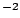to the NAMD units kcal/molÅamu, and print some information:  set linaccel_namd [vecscale [expr 1.0/418.68]$linaccel] print "Linear acceleration imparted: ($linaccel) Ang*ps^ -2" Note that to print messages from the force script, we must use the print command, instead of the usual puts command.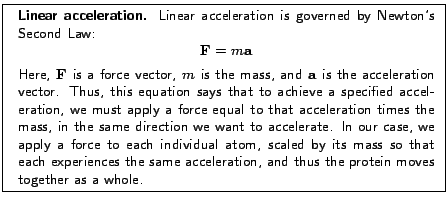5 The only thing left is the calcforces command:  proc calcforces { } { global atoms numatoms linaccel_namd loadcoords coords loadmasses masses set comsum "0.0 0.0 0.0" set totalmass 0.0 foreach atom$atoms { set force [vecscale $masses($atom) $linaccel_namd] addforce$atom $force set tmp [vecscale$masses($atom)$coords($atom)] set comsum [vecadd$comsum $tmp] } set invmass [expr 1.0/$totalmass] print "Center of mass = [vecscale $invmass$comsum]" }

The script starts with the global command, which imports the variables named so that they can be accessed in the calcforces command.

Next we call the command loadcoords. This sets the variable coords to a Tcl array of coordinates of the atoms that have been added using addatom. In this case, of course, that is all the atoms of the system. Array elements in Tcl are accessed using parentheses. Similarly, loadmasses retrieves the atomic masses, putting them in an array named masses.

Then we loop through the atoms, applying the specified force to each using the addforce command. Along the way, we also calculate the center of mass of the protein. We see two more Tcl commands in actions here: vecadd and vecscale. These add two vectors and multiply a vector by a scalar, respectively.

6
Because the system is so small, you can run it on your desktop or laptop without trouble, and see the protein move. Run the file push.namd. With a normal installation, you would do this with the command

namd2 push.namd > push.log7
After this finishes, we want to see how the protein was affected. We will now use VMD's MultiPlot plugin to view the results. Open the file plot.tcl in your favorite text editor, and change the first line to be the location of the log file you just created, e.g.

set log push.log

if you named the log file push.log. To run the script, either type

vmd -e plot.tcl

from the command line, or open VMD and source the script file in the Tk Console:

source plot.tcl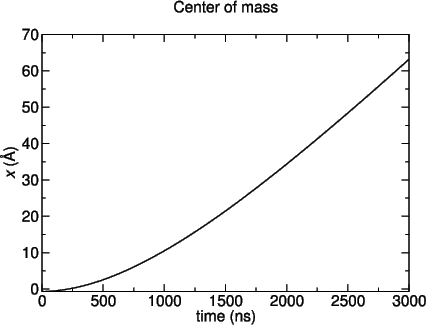8
You should see a plot similar to Fig. 2. The beginning of the graph is quadratic, as we expect. However, the velocity saturates at some value because of the Langevin dynamics used in the simulation. Langevin dynamics involve a damping factor, which creates a terminal velocity.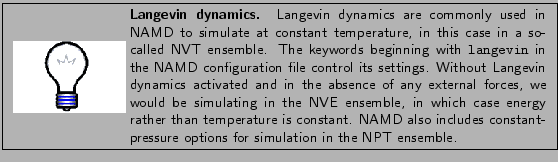9
Now open the trajectory in VMD. First launch VMD, then open the Tk Console. Make sure you're in the tclForcesFiles directory, then type the command

mol load psf common/ubiquitin.psf dcd push/push.dcd

If you could not produce the trajectory, there is a sample one in the example-output directory. Notice that the Langevin dynamics prevent the protein from moving too quickly.

Example 2: Rotation

We will now add a layer of complexity: we will force atoms differently based on their coordinates. In this example, we will force atoms in such a way that, in addition to a linear force, the protein also rotates about an axis parallel to the z-axis and through the protein's center of mass.

1
Change to the directory forces-tutorial-files/tclForcesFiles/rot-a.

2
Look at the NAMD configuration file. Open the file rot-a.namd. At the end, we see the following:

 tclforces on set linaccel "30 0 0" set angaccel 1 tclforcesscript rot-a.tcl

This is very similar to the previous example, but here we provide an additional scalar to describe the angular acceleration.

3
Now let's look at the script itself. Open the file rot-a.tcl. The atom selection part at the beginning is identical to the last case, so we will not repeat the discussion. The next part of the file is again similar, but also processes the angular acceleration.

 set linaccel_namd [vecscale [expr 1.0/418.68] $linaccel] set angaccel_namd [expr$angaccel/418.68]

 print "Linear acceleration: ($linaccel) Ang*ps^ -2" print "Angular acceleration: (0 0$angaccel) Rad*ps^ -2"

We convert to NAMD units, just like before. Then we print some informational text using the print command.

4
Now we'll look at the calcforces command. The first part is nearly the same as in the previous example. The differences are the change of one of the global variables, and the fact that we no longer apply forces in the first loop.

 proc calcforces { } { global atoms numatoms linaccel_namd angaccel_namd

 set comsum "0 0 0" set totalmass 0 foreach atom $atoms { set tmp [vecscale$masses($atom)$coords($atom)] set comsum [vecadd$comsum $tmp set totalmass [expr$totalmass + $masses($atom)] } set com [vecscale [expr 1.0/$totalmass]$comsum] print "Center of mass = $com" 5 The last part of the script is where the real differences lie:  foreach atom$atoms { set linforce [vecscale $masses($atom) $linaccel_namd] set r [vecsub$coords($atom)$com] set x [lindex $r 0] set y [lindex$r 1] set rho [expr sqrt($x*$x + $y*$y)] set phi [expr atan2($y,$x) + $M_PI/2]  if {$atom == 1 } { print "atom $atom: phi =$phi" }

 set angdir "[expr cos($phi)] [expr sin($phi)] 0.0" set angmag [expr $masses($atom) * $angaccel_namd *$rho] set angforce [vecscale $angmag$angdir] set force [vecadd $linforce$angforce] addforce $atom$force } }

The first section above calculates the distance from the rotation axis, rho, and the angle from the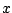axis at which the force should be applied, phi (from the angle of the position vector, hence the addition ofradians.) Fig. 3 shows how these variables are related.After printing the force angle associated with one atom so we can monitor the rotation, we find a unit vector (i.e. a vector with length one) pointing in the phi direction. We then multiply this by the mass of the atom and its distance from our rotation axis, giving us the correct angular force for the angular acceleration requested in the NAMD configuration file. Finally, we add the linear and angular forces to get the total force.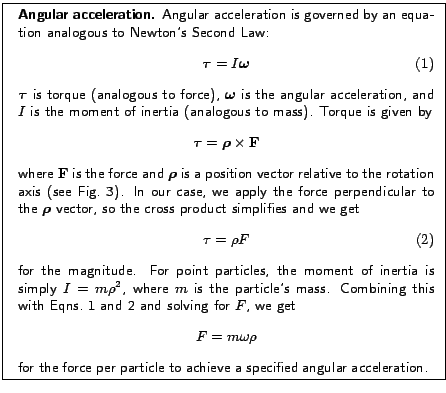6
Now run the simulation:

namd2 rot-a.namd > rot-a.log

This should again take just a few minutes.

7
Plot the angle phi by using the script plot.tcl just as you did before (there is a different copy in the current directory.)

The result is shown in Fig. 4. Plot the center of mass as well, by opening plot.tcl and changing the var variable to "Center of mass". This can be accomplished by simply commenting the first set var line, and uncommenting the other:

 #set var "atom 1: phi" set var "Center of mass"8
We again see a roughly quadratic time dependence at the beginning of the trajectory give way to a linear one, again a consequence of the Langevin damping.

9
Open the trajectory in VMD. With VMD open, go to the main tclForcesFiles directory, then type the following in the Tk Console:

mol load psf common/ubiquitin.psf dcd rot-a/rot-a.dcd

Challenge: Find the dependence of the terminal linear and angular velocities on the langevinDamping parameter of the simulation, set in the configuration file, as well as the dependence on the applied force.

Example 3: Forcing a Subset of Atoms

This example deals with the important ability to force a subset of your atoms. In this section, we will apply forces only to the backbone atoms of the protein. We accomplish this by making a special target atom'' PDB file, using the beta column to mark the atoms we want to apply force to. In addition, we will use the occupancy column to tell our script the atomic masses, in order to demonstrate how to provide the script with additional information.

1
Change to the directory forces-tutorial-files/tclForcesFiles/rot-b.

2
As usual, we will first examine the NAMD configuration file. Open the file rot-b-short.namd. First, note that we are now using a different system, one in which the ubiquitin has been solvated. This is a more natural environment for the protein.

Look at the end of the file, where we set up tclForces. We see a new line:

set targetAtomPdb ../common/ubiquitin_solvate_backbone.pdb

With this line, we specify the aforementioned target atom PDB file. Thus, our first task is to produce that file. We will use a VMD script to accomplish this.

3
Open the file makeTargetAtomPdb.tcl. The first section sets a few variables. First, the relevant file names:

 set pdb common/ubiquitin_solvate.pdb set psf common/ubiquitin_solvate.psf set targetPdb common/ubiquitin_solvate_backbone.pdb

Next, we set the selection text we will use to select our atoms, and the beta value that these atoms will receive:

 set selection "protein and backbone" set targetMark "1.00"

4
Next, we load the structure into VMD, and set both the beta and occupancy columns to zero:

 mol load psf $psf pdb$pdb set all [atomselect top all] $all set beta 0$all set occupancy 0

5
Now we make a selection of the target atoms, set their beta and occupancy columns to the appropriate values, and write the output PDB. Note the ease with which the list of masses are used to set the occupancy:

 set target [atomselect top $selection] set masses [$target get mass] $target set beta$targetMark $target set occupancy$masses

 $all writepdb$targetPdb exit

6
Now run the script:

vmd -dispdev text -e makeTargetAtomPdb.tcl

(Note: For Windows users, open the VMD GUI and source the script file in the Tk Console.)

If you were unable to run the script, copy the file example-output/
ubiquitin_solvate_backbone.pdb
into the common directory.

7
Next, take a look at the tclForces script. Open the file rot-b.tcl. Right from the beginning, we notice many differences. It first sets a targetMark variable so that the script can recognize the marks we've set in the target PDB:

set targetMark 1.0

8
Now we have to process the PDB. There are no built-in routines for accomplishing this, so the following code is quite low-level. The columns of a PDB file have a fixed character width, so we must simply read each line, and break it into fixed-sized pieces according to the PDB format:

 set targets {} set masses {} set inStream [open $targetAtomPdb r] foreach line [split [read$inStream]n] { set type [string trim [string range $line 0 5]] set name [string trim [string range$line 12 15]] set resid [string trim [string range $line 22 25]] set beta [string trim [string range$line 60 65]] set occupancy [string trim [string range $line 54 59]] set segname [string trim [string range$line 72 75]]

9
We first make sure that this line corresponds to an atom record and not a comment line or some other entry. Then, if the atom has a beta value matching the target value, we form a triple consisting of the atom's segname, resid, and name. This is necessary for finding the index of the atom:

 if { ($type eq "ATOM" ||$type eq "HETATM") \ && $beta ==$targetMark } { lappend targets "$segname$resid $name" lappend masses$occupancy } } close $inStream 10 The next step is to use the atom triples to find its index, and form a list of these, using the atomindex command. addatom is then called for each of these atoms:  set atoms {} foreach target$targets { lassign $target segname resid atom set atomindex [atomid$segname $resid$atom] lappend atoms $atomindex addatom$atomindex }

11
Next we find the number of target atoms:

 set numatoms [llength $atoms] set linaccel_namd [vecscale [expr 1.0/418.68]$linaccel] set angaccel_namd [expr $angaccel/418.68] print "Linear acceleration: ($linaccel) Ang*ps^ -2" print "Angular acceleration: (0 0 $angaccel) Rad*ps^ -2" 12 Now we get to the main part of the script, the calcforces definition. It is essentially identical to the last example:  proc calcforces { } { global atoms numatoms masses linaccel_namd angaccel_namd  loadcoords coords set comsum "0 0 0" set totalmass 0 foreach atom$atoms mass $masses { set tmp [vecscale$mass $coords($atom)] set comsum [vecadd $comsum$tmp] set totalmass [expr $totalmass +$mass] } set com [vecscale [expr 1.0/$totalmass]$coordsum] print "Center = $com"  foreach atom$atoms mass $masses { set linforce [vecscale$mass $linaccel_namd] set r [vecsub$coords($atom)$com] set x [lindex $r 0] set y [lindex$r 1] set rho [expr sqrt($x*$x + $y*$y)] set phi [expr atan2($y,$x) + $M_PI/2]  if {$atom == 1 } { print "atom $atom: phi =$phi" }

 set angdir "[expr cos($phi)] [expr sin($phi)] 0.0" set angmag [expr $angaccel_namd *$rho * $mass] set angforce [vecscale$angmag $angdir] set force [vecadd$linforce $angforce] addforce$atom $force } } 13 Now run the simulation, just as you have in previous examples: namd2 rot-b-short.namd > rot-b-short.log If you have access to a cluster, run it on the cluster--refer to local instructions for how this is done. This simulation will run for just 100 steps, so that the benchmark times are printed. In the next section, we will see how to improve this performance. If you are interested, examine the files rot-b-long.log and rot-b-long.dcd in the example-output directory, which were produced with a slightly longer simulation. When loading the trajectory in VMD, use the PSF common/ubiquitin_solvate.psf Example 4: Improving Efficiency In most cases, it's unnecessary to recalculate the force values applied at each time step. Depending on the situation, one may be able to recalculate every 1000 steps, saving a lot of computational effort and therefore increasing the speed of the simulation. In this section, we will see how to do that. 1 Change to the directory forces-tutorial-files/tclForcesFiles/rot-c. 2 Open the NAMD configuration file, rot-c-short.namd. The new line this time is set forcesRecalcFreq 10 As its name implies, this parameter sets how often our forces will be recalculated. 3 Now open the script rot-c.tcl. The first differences start at line 58. We first set up a forces list, which is a variable to hold the values of the forces we will apply to the atoms. We also set up some counter variables: forcecount and printcount are both incremented each timestep. When forcecount equals forcesRecalcFreq, the forces are recalculated, and forcecount is reset to zero, as seen below.  set forces {} foreach index$atoms { lappend forces "0.0 0.0 0.0" }

 set forcecount $forcesRecalcFreq set printcount 0 4 Now we begin the calcforces command. First, as usual, we declare our global variables. We then apply the forces that are saved in the forces list.  proc calcforces { } { global atoms numatoms forcemult masses avgmass forces global forcesRecalcFreq global forcecount printcount  foreach atom$atoms force $forces { addforce$atom $force } 5 Next, we test whether the forces will be recalculated next timestep, and if so, tell NAMD that we will want atomic coordinates for the target atoms by calling addatom:  if {$forcecount == [expr $forcesRecalcFreq - 1] } { print "Adding atoms prior to reconfiguring forces at$printcount" foreach atom $atoms { addatom$atom } }

As we will see shortly, we call clearconfig after the forces are recalculated. This call erases all addatom records; without it, the coordinates of the atoms added will be available every timestep, independently of whether we call loadcoords, and therefore much of the potential speed gain will be lost. However, this means that addatom must be called again each time we want to recalculate forces, and because of technical details it has to be done at least one step before the coordinates will be used.

6
Next we have the code that recalculates the force. As alluded to above, the recalculation happens when forcecount equals forcesRecalcFreq. The rest of the force calculation code is identical to the code we had in the last example, except that instead of calling addforce with each force vector, we now put the vector in the forces list variable:

 if { $forcecount ==$forcesRecalcFreq } { print "Recalculating forces at $printcount"  loadcoords coords set comsum "0 0 0" set totalmass 0 foreach atom$atoms mass $masses { set tmp [vecscale$mass $coords($atom)] set comsum [vecadd $comsum$tmp] set totalmass [expr $totalmass +$mass] } set com [vecscale [expr 1.0/$totalmass]$comsum] print "Center of mass = $com"  set forces {} foreach atom$atoms mass $masses { set linforce [vecscale$mass $linaccel_namd] set r [vecsub$coords($atom)$com] set x [lindex $r 0] set y [lindex$r 1] set rho [expr sqrt($x*$x + $y*$y)] set phi [expr atan2($y,$x) + $M_PI/2]  if {$atom == 1 } { print "atom $atom: phi =$phi" }

 set angdir "[expr cos($phi)] [expr sin($phi)] 0.0" set angmag [expr $angaccel_namd *$rho * $mass] set angforce [vecscale$angdir] set force [vecadd $linforce$angforce] lappend forces $force } 7 Finally, we print some information, call clearconfig, and reset forcecount.  print "Step${printcount}: Recalculated[llength \$forces] forces" set forcecount 0 clearconfig } incr forcecount incr printcount return }

8
Now run the simulation:

namd2 rot-c-short.namd > rot-c-short.log

Again, this is a short simulation, running 100 steps, just long enough for benchmark timing information to be printed. Compare the speed of this simulation to the last. There should be a significant difference--comparing the example files rot-b-short.log and rot-c-short.log, which were run on just a single CPU, there is nearly a 15% speed increase. As the size of the system and the number of CPUs increase, applying tclForces efficiently becomes even more important: comparing rot-c-long.log with rot-b-long.log, both run on 20 processors, rot-c is almost 60% faster!

Once again, there are sample output files, rot-c-long.dcd and rot-c-long.log in the example-output directory, and should again be loaded together with the PSF file common/ubiquitin_solvate.psf.

Challenge: Take the provided file kcsa_closed.pdb, and use the partial structure kcsa_open.pdb as a target to open the channel using tclForces.

You now know the basics of tclForces. In the next section, you will learn about a similar feature of NAMD called tclBC, which is suitable for different circumstances.Next: TclBC Up: User-Defined Forces in NAMD Previous: Introduction
tutorial-l@ks.uiuc.edu